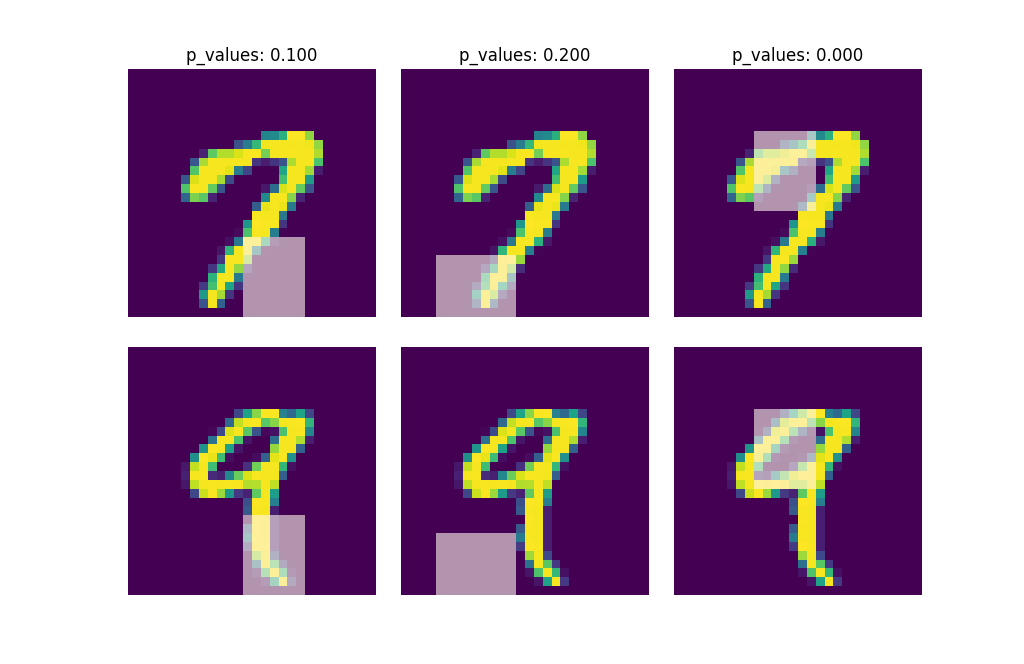# 🔬 dnn-inference¶dnn-inference is a Python module for hypothesis testing based on blackbox models, including deep neural networks.

## 🎯 What We Can Do¶dnn-inference is able to provide an asymptotically valid p-value to examine if $$\mathcal{S}$$ is discriminative features to predict $$Y$$. Specifically, the proposed testing is:

$H_0: R(f^*) - R_{\mathcal{S}}(g^*) = 0, \quad \text{versus} \quad H_a: R(f^*) - R_{\mathcal{S}}(g^*) < 0,$

where $$\mathcal{S}$$ is a collection of hypothesized features, $$R$$ and $$R_{\mathcal{S}}$$ are risk functions with/without the hypothesized features $$\mathbf{X}_{\mathcal{S}}$$, and $$f^*$$ and $$g^*$$ are population minimizers on $$R$$ and $$R_{\mathcal{S}}$$ respectively. The proposed test just considers the difference between the best predictive scores with/without hypothesized features. Please check more details in our paper arXiv:2103.04985.

• When log-likelihood is used as a loss function, then the test is equivalent to a conditional independence test: $$Y \perp X_{\mathcal{S}} | X_{\mathcal{S}^c}$$.

• Only a small number of fitting on neural networks is required, and the number can be as small as 1.

• Asymptotically Type I error control and power consistency.

## Reference¶

If you use this code please star the repository and cite the following paper:

@misc{dai2021significance,
title={Significance tests of feature relevance for a blackbox learner},
author={Ben Dai and Xiaotong Shen and Wei Pan},
year={2021},
eprint={2103.04985},
archivePrefix={arXiv},
primaryClass={stat.ML}
}